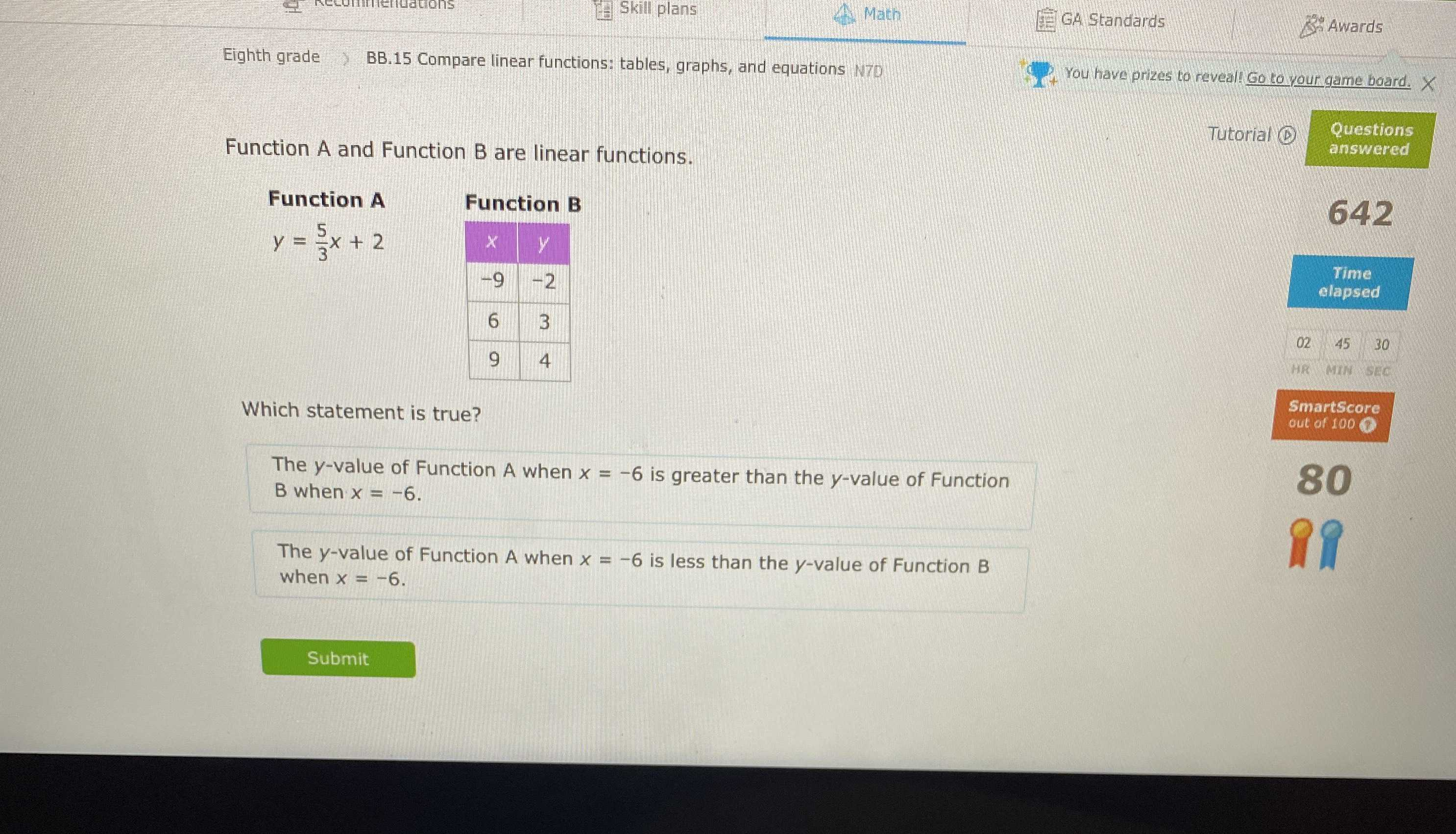### Still have math questions?

Algebra
QuestionFunction A and Function B are linear functions.

Function $$A$$ : $$y = \frac { 5 } { 3 } x + 2$$

Function $$B$$ : $$\begin{array}{|c|c|c|c|} \hline x&- 9&6&9\\ \hline y&- 2&3&4\\ \hline \end{array}$$

Which statement is true?

(A). The $$y$$ -value of Function A when $$x = - 6$$ is greater than the $$y$$ -value of Function B when $$x = - 6$$

(B). The $$y$$ -value of Function A when $$x = - 6$$ is less than the $$y$$ -value of Function B when $$x = - 6$$ .